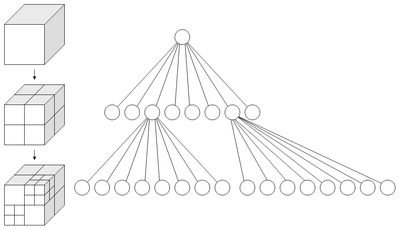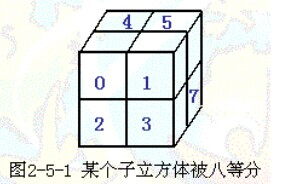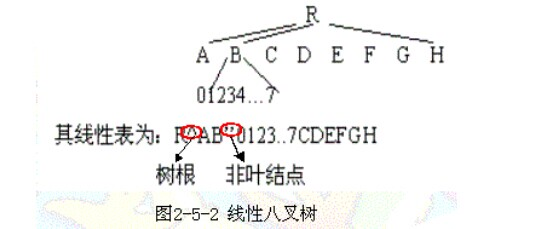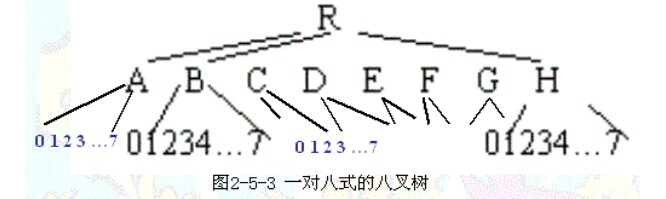# 八叉树Octree八叉树是一种用于描述三维空间的树状数据结构。八叉树的每个节点表示一个正方体的体积元素，每个节点有八个子节点，将八个子节点所表示的体积元素加在一起就等于父节点的体积。

(1). 设定最大递归深度。

(2). 找出场景的最大尺寸，并以此尺寸建立第一个立方体。

(3). 依序将单位元元素丢入能被包含且没有子节点的立方体。

(4). 若没达到最大递归深度，就进行细分八等份，再将该立方体所装的单位元元素全部分担给八个子立方体。

(5). 若发现子立方体所分配到的单位元元素数量不为零且跟父立方体是一样的，则该子立方体停止细分，因为跟据空间分割理论，细分的空间所得到的分配必定较少，若是一样数目，则再怎么切数目还是一样，会造成无穷切割的情形。

(6). 重复3，直到达到最大递归深度。

八叉树三维数据结构

（一）   基本原理另外，由于这种方法充分利用了形体在空上的相关性，因此，一般来说，它所占用的存贮空间要比三维体素阵列的少。但是实际上它还是使用了相当多的存贮，这并不是八叉树的主要优点。这一方法的主要优点在于可以非常方便地实现有广泛用途的集合运算(例如可以求两个物体的并、交、差等运算)，而这些恰是其它表示方法比较难以处理或者需要耗费许多计算资源的地方。不仅如此，由于这种方法的有序性及分层性，因而对显示精度和速度的平衡、隐线和隐面的消除等，带来了很大的方便，特别有用。

（二）八叉树的存贮结构

1、规则八叉树

规则八叉树的存贮结构用一个有九个字段的记录来表示树中的每个结点。其中一个字段用来描述该结点的特性(在目前假定下，只要描述它是灰、白、黑三类结点中哪一类即可)，其余的八个字段用来作为存放指向其八个子结点的指针。这是最普遍使用的表示树形数据的存贮结构方式。

规则八叉树缺陷较多，最大的问题是指针占用了大量的空间。假定每个指针要用两个字节表示，而结点的描述用一个字节，那么存放指针要占总的存贮量的94％。因此，这种方法虽然十分自然，容易掌握，但在存贮空间的使用率方面不很理想。

2、线性八叉树

线性八叉树注重考虑如何提高空间利用率。用某一预先确定的次序遍历八叉树(例如以深度第一的方式)，将八叉树转换成一个线性表（图2-5-2），表的每个元素与一个结点相对应。对于结点的描述可以丰富一点，例如用适当的方式来说明它是否为叶结点，如果不是叶结点时还可用其八个子结点值的平均值作为非叶结点的值等等。这样，可以在内存中以紧凑的方式来表示线性表，可以不用指针或者仅用一个指针表示即可。3、一对八式的八叉树

一个非叶结点有八个子结点，为了确定起见，将它们分别标记为0，1，2，3，4，5，6，7。从上面的介绍可以看到，如果一个记录与一个结点相对应，那么在这个记录中描述的是这个结点的八个子结点的特性值。而指针给出的则是该八个子结点所对应记录的存放处，而且还隐含地假定了这些子结点记录存放的次序。也就是说，即使某个记录是不必要的(例如，该结点已是叶结点)，那么相应的存贮位置也必须空闲在那里（图2-5-3），以保证不会错误地存取到其它同辈结点的记录。这样当然会有一定的浪费，除非它是完全的八叉树，即所有的叶结点均在同一层次出现，而在该层次之上的所有层中的结点均为非叶结点。http://wenku.baidu.com/link?url=oFbVyAmf0P04HF04g6DSZo6c3HbGvnNCZ9sErgh9QM04Y11pXtId8F-SccwKnLlS86LnHZLr-1UUClcBM8Ycjof6PE4WHi2g0dKGMTQ4nRi

(1). 设定最大递归深度
(2). 找出场景的最大尺寸，并以此尺寸建立第一个立方体
(3). 依序将单位元元素丢入能被包含且没有子节点的立方体
(4). 若没有达到最大递归深度，就进行细分八等份，再将该立方体所装的单位元元素全部分担给八个子立方体
(5). 若发现子立方体所分配到的单位元元素数量不为零且跟父立方体是一样的，则该子立方体停止细分，因为跟据空间分割理论，细分的空间所得到的分配必定较少，若是一样数目，则再怎么切数目还是一样，会造成无穷切割的情形。
(6). 重复3，直到达到最大递归深度。

#include <iostream>

using namespace std;
//定义八叉树节点类
template<class T>
struct OctreeNode
{
T data; //节点数据
T xmin,xmax; //节点坐标，即六面体个顶点的坐标
T ymin,ymax;
T zmin,zmax;
OctreeNode <T>*top_left_front,*top_left_back; //该节点的个子结点
OctreeNode <T>*top_right_front,*top_right_back;
OctreeNode <T>*bottom_left_front,*bottom_left_back;
OctreeNode <T>*bottom_right_front,*bottom_right_back;
OctreeNode //节点类
(T nodeValue = T(),
T xminValue = T(),T xmaxValue = T(),
T yminValue = T(),T ymaxValue = T(),
T zminValue = T(),T zmaxValue = T(),
OctreeNode<T>*top_left_front_Node = NULL,
OctreeNode<T>*top_left_back_Node = NULL,
OctreeNode<T>*top_right_front_Node = NULL,
OctreeNode<T>*top_right_back_Node = NULL,
OctreeNode<T>*bottom_left_front_Node = NULL,
OctreeNode<T>*bottom_left_back_Node = NULL,
OctreeNode<T>*bottom_right_front_Node = NULL,
OctreeNode<T>*bottom_right_back_Node = NULL )
:data(nodeValue),
xmin(xminValue),xmax(xmaxValue),
ymin(yminValue),ymax(ymaxValue),
zmin(zminValue),zmax(zmaxValue),
top_left_front(top_left_front_Node),
top_left_back(top_left_back_Node),
top_right_front(top_right_front_Node),
top_right_back(top_right_back_Node),
bottom_left_front(bottom_left_front_Node),
bottom_left_back(bottom_left_back_Node),
bottom_right_front(bottom_right_front_Node),
bottom_right_back(bottom_right_back_Node){}
};
//创建八叉树
template <class T>
void createOctree(OctreeNode<T> * &root,int maxdepth,double xmin,double xmax,double ymin,double ymax,double zmin,double zmax)
{
//cout<<"处理中，请稍候……"<<endl;
maxdepth=maxdepth-1; //每递归一次就将最大递归深度-1
if(maxdepth>=0)
{
root=new OctreeNode<T>();
cout<<"请输入节点值:";
//root->data =9;//为节点赋值，可以存储节点信息，如物体可见性。由于是简单实现八叉树功能，简单赋值为9。
cin>>root->data;  //为节点赋值
root->xmin=xmin; //为节点坐标赋值
root->xmax=xmax;
root->ymin=ymin;
root->ymax=ymax;
root->zmin=zmin;
root->zmax=zmax;
double xm=(xmax-xmin)/2;//计算节点个维度上的半边长
double ym=(ymax-ymin)/2;
double zm=(ymax-ymin)/2;
//递归创建子树，根据每一个节点所处（是几号节点）的位置决定其子结点的坐标。
createOctree(root->top_left_front,maxdepth,xmin,xmax-xm,ymax-ym,ymax,zmax-zm,zmax);
createOctree(root->top_left_back,maxdepth,xmin,xmax-xm,ymin,ymax-ym,zmax-zm,zmax);
createOctree(root->top_right_front,maxdepth,xmax-xm,xmax,ymax-ym,ymax,zmax-zm,zmax);
createOctree(root->top_right_back,maxdepth,xmax-xm,xmax,ymin,ymax-ym,zmax-zm,zmax);
createOctree(root->bottom_left_front,maxdepth,xmin,xmax-xm,ymax-ym,ymax,zmin,zmax-zm);
createOctree(root->bottom_left_back,maxdepth,xmin,xmax-xm,ymin,ymax-ym,zmin,zmax-zm);
createOctree(root->bottom_right_front,maxdepth,xmax-xm,xmax,ymax-ym,ymax,zmin,zmax-zm);
createOctree(root->bottom_right_back,maxdepth,xmax-xm,xmax,ymin,ymax-ym,zmin,zmax-zm);
}
}
int i=1;
//先序遍历八叉树
template <class T>
void preOrder( OctreeNode<T> * & p)
{
if(p)
{
cout<<i<<".当前节点的值为："<<p->data<<"\n坐标为：";
cout<<"xmin: "<<p->xmin<<" xmax: "<<p->xmax;
cout<<"ymin: "<<p->ymin<<" ymax: "<<p->ymax;
cout<<"zmin: "<<p->zmin<<" zmax: "<<p->zmax;
i+=1;
cout<<endl;
preOrder(p->top_left_front);
preOrder(p->top_left_back);
preOrder(p->top_right_front);
preOrder(p->top_right_back);
preOrder(p->bottom_left_front);
preOrder(p->bottom_left_back);
preOrder(p->bottom_right_front);
preOrder(p->bottom_right_back);
cout<<endl;
}
}
//求八叉树的深度
template<class T>
int depth(OctreeNode<T> *& p)
{
if(p == NULL)
return -1;
int h =depth(p->top_left_front);
return h+1;
}
//计算单位长度，为查找点做准备
int cal(int num)
{
int result=1;
if(1==num)
result=1;
else
{
for(int i=1;i<num;i++)
result=2*result;
}
return result;
}
//查找点
int maxdepth=0;
int times=0;
static double xmin=0,xmax=0,ymin=0,ymax=0,zmin=0,zmax=0;
int tmaxdepth=0;
double txm=1,tym=1,tzm=1;
template<class T>
void find(OctreeNode<T> *& p,double x,double y,double z)
{
double xm=(p->xmax-p->xmin)/2;
double ym=(p->ymax-p->ymin)/2;
double zm=(p->ymax-p->ymin)/2;
times++;
if(x>xmax || x<xmin|| y>ymax || y<ymin || z>zmax || z<zmin)
{
cout<<"该点不在场景中！"<<endl;
return;
}
if(x<=p->xmin+txm&& x>=p->xmax-txm && y<=p->ymin+tym &&y>=p->ymax-tym && z<=p->zmin+tzm &&z>=p->zmax-tzm )
{
cout<<endl<<"找到该点！"<<"该点位于"<<endl;
cout<<"xmin: "<<p->xmin<<" xmax: "<<p->xmax;
cout<<"ymin: "<<p->ymin<<" ymax: "<<p->ymax;
cout<<"zmin: "<<p->zmin<<" zmax: "<<p->zmax;
cout<<"节点内！"<<endl;
cout<<"共经过"<<times<<"次递归！"<<endl;
}
else if(x<(p->xmax-xm) && y<(p->ymax-ym) &&z<(p->zmax-zm))
{
cout<<"当前经过节点坐标："<<endl;
cout<<"xmin: "<<p->xmin<<" xmax: "<<p->xmax;
cout<<"ymin: "<<p->ymin<<" ymax: "<<p->ymax;
cout<<"zmin: "<<p->zmin<<" zmax: "<<p->zmax;
cout<<endl;
find(p->bottom_left_back,x,y,z);
}
else if(x<(p->xmax-xm) && y<(p->ymax-ym) &&z>(p->zmax-zm))
{
cout<<"当前经过节点坐标："<<endl;
cout<<"xmin: "<<p->xmin<<" xmax: "<<p->xmax;
cout<<"ymin: "<<p->ymin<<" ymax: "<<p->ymax;
cout<<"zmin: "<<p->zmin<<" zmax: "<<p->zmax;
cout<<endl;
find(p->top_left_back,x,y,z);
}
else if(x>(p->xmax-xm) && y<(p->ymax-ym) &&z<(p->zmax-zm))
{
cout<<"当前经过节点坐标："<<endl;
cout<<"xmin: "<<p->xmin<<" xmax: "<<p->xmax;
cout<<"ymin: "<<p->ymin<<" ymax: "<<p->ymax;
cout<<"zmin: "<<p->zmin<<" zmax: "<<p->zmax;
cout<<endl;
find(p->bottom_right_back,x,y,z);
}
else if(x>(p->xmax-xm) && y<(p->ymax-ym) &&z>(p->zmax-zm))
{
cout<<"当前经过节点坐标："<<endl;
cout<<"xmin: "<<p->xmin<<" xmax: "<<p->xmax;
cout<<"ymin: "<<p->ymin<<" ymax: "<<p->ymax;
cout<<"zmin: "<<p->zmin<<" zmax: "<<p->zmax;
cout<<endl;
find(p->top_right_back,x,y,z);
}
else if(x<(p->xmax-xm) && y>(p->ymax-ym) &&z<(p->zmax-zm))
{
cout<<"当前经过节点坐标："<<endl;
cout<<"xmin: "<<p->xmin<<" xmax: "<<p->xmax;
cout<<"ymin: "<<p->ymin<<" ymax: "<<p->ymax;
cout<<"zmin: "<<p->zmin<<" zmax: "<<p->zmax;
cout<<endl;
find(p->bottom_left_front,x,y,z);
}
else if(x<(p->xmax-xm) && y>(p->ymax-ym) &&z>(p->zmax-zm))
{
cout<<"当前经过节点坐标："<<endl;
cout<<"xmin: "<<p->xmin<<" xmax: "<<p->xmax;
cout<<"ymin: "<<p->ymin<<" ymax: "<<p->ymax;
cout<<"zmin: "<<p->zmin<<" zmax: "<<p->zmax;
cout<<endl;
find(p->top_left_front,x,y,z);
}
else if(x>(p->xmax-xm) && y>(p->ymax-ym) &&z<(p->zmax-zm))
{
cout<<"当前经过节点坐标："<<endl;
cout<<"xmin: "<<p->xmin<<" xmax: "<<p->xmax;
cout<<"ymin: "<<p->ymin<<" ymax: "<<p->ymax;
cout<<"zmin: "<<p->zmin<<" zmax: "<<p->zmax;
cout<<endl;
find(p->bottom_right_front,x,y,z);
}
else if(x>(p->xmax-xm) && y>(p->ymax-ym) &&z>(p->zmax-zm))
{
cout<<"当前经过节点坐标："<<endl;
cout<<"xmin: "<<p->xmin<<" xmax: "<<p->xmax;
cout<<"ymin: "<<p->ymin<<" ymax: "<<p->ymax;
cout<<"zmin: "<<p->zmin<<" zmax: "<<p->zmax;
cout<<endl;
find(p->top_right_front,x,y,z);
}
}
//main函数
int main ()
{
OctreeNode<double> *rootNode = NULL;
int choiced = 0;
while(true)
{
system("cls");
cout<<"请选择操作：\n";
cout<<"1.创建八叉树 2.先序遍历八叉树\n";
cout<<"3.查看树深度 4.查找节点   \n";
cout<<"0.退出\n\n";
cin>>choiced;
if(choiced == 0)
return 0;
else if(choiced == 1)
{
system("cls");
cout<<"请输入最大递归深度："<<endl;
cin>>maxdepth;
cout<<"请输入外包盒坐标，顺序如下：xmin,xmax,ymin,ymax,zmin,zmax"<<endl;
cin>>xmin>>xmax>>ymin>>ymax>>zmin>>zmax;
if(maxdepth>=0|| xmax>xmin || ymax>ymin || zmax>zmin || xmin>0 || ymin>0||zmin>0)
{
tmaxdepth=cal(maxdepth);
txm=(xmax-xmin)/tmaxdepth;
tym=(ymax-ymin)/tmaxdepth;
tzm=(zmax-zmin)/tmaxdepth;
createOctree(rootNode,maxdepth,xmin,xmax,ymin,ymax,zmin,zmax);
}
else
{
cout<<"输入错误！";
return 0;
}
}
else if(choiced == 2)
{
system("cls");
cout<<"先序遍历八叉树结果：/n";
i=1;
preOrder(rootNode);
cout<<endl;
system("pause");
}
else if(choiced == 3)
{
system("cls");
int dep =depth(rootNode);
cout<<"此八叉树的深度为"<<dep+1<<endl;
system("pause");
}
else if(choiced == 4)
{
system("cls");
cout<<"请输入您希望查找的点的坐标，顺序如下：x,y,z\n";
double x,y,z;
cin>>x>>y>>z;
times=0;
cout<<endl<<"开始搜寻该点……"<<endl;
find(rootNode,x,y,z);
system("pause");
}
else
{
system("cls");
cout<<"\n\n错误选择！\n";
system("pause");
}
}
}

http://blog.csdn.net/chshji666666/article/details/5841032